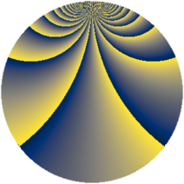# Properties

 Label 1470.2.bmLevel $1470$ Weight $2$ Character orbit 1470.bm Rep. character $\chi_{1470}(59,\cdot)$ Character field $\Q(\zeta_{42})$ Dimension $1344$ Sturm bound $672$

# Related objects

## Defining parameters

 Level: $$N$$ $$=$$ $$1470 = 2 \cdot 3 \cdot 5 \cdot 7^{2}$$ Weight: $$k$$ $$=$$ $$2$$ Character orbit: $$[\chi]$$ $$=$$ 1470.bm (of order $$42$$ and degree $$12$$) Character conductor: $$\operatorname{cond}(\chi)$$ $$=$$ $$735$$ Character field: $$\Q(\zeta_{42})$$ Sturm bound: $$672$$

## Dimensions

The following table gives the dimensions of various subspaces of $$M_{2}(1470, [\chi])$$.

Total New Old
Modular forms 4128 1344 2784
Cusp forms 3936 1344 2592
Eisenstein series 192 0 192

## Trace form

 $$1344q + 112q^{4} + 14q^{6} - 16q^{9} + O(q^{10})$$ $$1344q + 112q^{4} + 14q^{6} - 16q^{9} + 6q^{10} - 12q^{15} + 112q^{16} + 24q^{19} - 10q^{21} + 6q^{24} - 2q^{25} + 6q^{30} + 12q^{31} - 10q^{36} + 20q^{39} + 22q^{40} + 120q^{45} - 156q^{46} + 72q^{49} + 12q^{51} + 322q^{55} + 6q^{60} - 52q^{61} - 224q^{64} - 48q^{66} + 24q^{70} + 96q^{75} + 28q^{79} - 48q^{81} + 68q^{84} + 16q^{85} + 42q^{90} - 128q^{91} + 16q^{94} - 6q^{96} - 192q^{99} + O(q^{100})$$

## Decomposition of $$S_{2}^{\mathrm{new}}(1470, [\chi])$$ into newform subspaces

The newforms in this space have not yet been added to the LMFDB.

## Decomposition of $$S_{2}^{\mathrm{old}}(1470, [\chi])$$ into lower level spaces

$$S_{2}^{\mathrm{old}}(1470, [\chi]) \cong$$ $$S_{2}^{\mathrm{new}}(735, [\chi])$$$$^{\oplus 2}$$